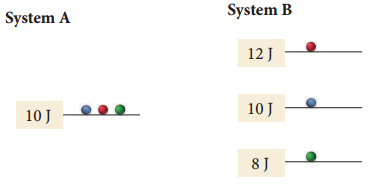# Problem: Two systems, each composed of three particles represented by circles, have 30 J of total energy.How many energetically equivalent ways can you distribute the particles in system B?

###### FREE Expert Solution
82% (192 ratings)
###### Problem Details
Two systems, each composed of three particles represented by circles, have 30 J of total energy.How many energetically equivalent ways can you distribute the particles in system B?